×#### Thank you for registering.

One of our academic counsellors will contact you within 1 working day.

Click to Chat

1800-1023-196

+91-120-4616500

CART 0

• 0

MY CART (5)

Use Coupon: CART20 and get 20% off on all online Study Material

ITEM
DETAILS
MRP
DISCOUNT
FINAL PRICE
Total Price: Rs.

There are no items in this cart.
Continue Shopping• Complete JEE Main/Advanced Course and Test Series
• OFFERED PRICE: Rs. 15,900
• View Details

```Revision Notes on Understanding Quadrilaterals

Plane Surface

A flat surface like paper is a plane surface.

Plane Curve

When we get a curve by joining the number of points without lifting the pencil is a plane curve.

It could be an open or closed curve.

Open Curve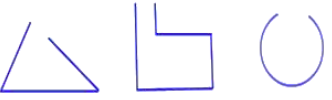Closed CurvePolygons

The simple closed curves which are made up of line segments only are called the Polygons.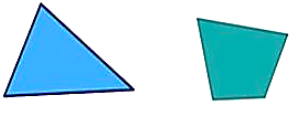Classification of Polygons

Polygons can be classified by the number of sides or vertices they have.

Number of sides
Name of Polygon
Figure

3
Triangle4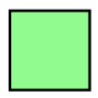5
Pentagon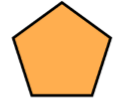6
Hexagon7
Heptagon8
Octagon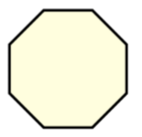9
Nonagon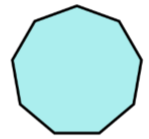10
Decagon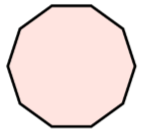n
n-gon

Diagonals

Any line segment which connects the two non-consecutive vertices of a polygon is called Diagonal.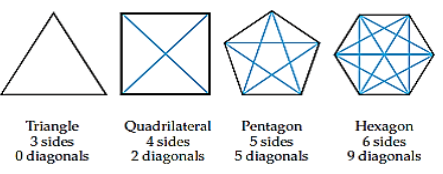Interior and Exterior of a Closed CurveThe blue shaded part represents the interior and exterior of the closed curve.

Convex and Concave Polygons

The polygons which have all the diagonals inside the figure are known as a Convex Polygon.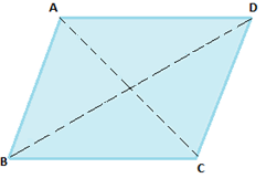The polygons which have some of its diagonals outside the figure also are known as a Concave Polygon.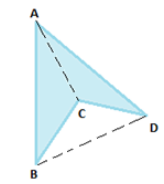Regular and Irregular Polygons

Polygons which are equiangular and equilateral are called Regular Polygons i.e. a polygon is regular if-

It’s all sides are equal.

It’s all angles are equal.Hence square is a regular polygon but a rectangle is not as its angles are equal but sides are not equal.

Angle Sum Property

The sum of all the interior angles of a polygon remains the same according to the number of sides regardless of the shape of the polygon.

The sum of interior angles of a polygon is-

(n - 2) × 180°

Where n = number of sides of the polygon

Example

Polygon
Number of Sides
Sum of Interior Angles

Triangle
3
(3 – 2) × 180° = 180°

4
(4 – 2)* × 180° = 360°

n-gon
n
(n – 2) × 180°

Remark: This property is applicable to both convex and concave polygon.

Sum of the Measures of the Exterior Angles of a Polygon

The sum of the exterior angles of any polygon will be 360°.

This is used to find the number of sides in a regular polygon.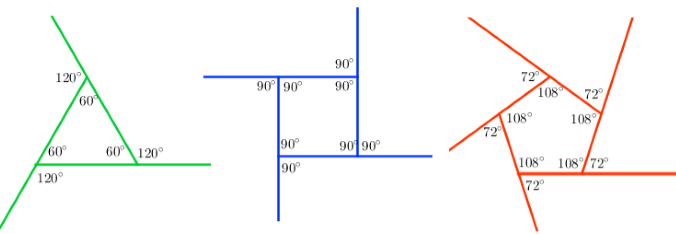This is applicable to irregular polygon also. The sum will remain the same whether it is a regular or irregular, small or large polygon.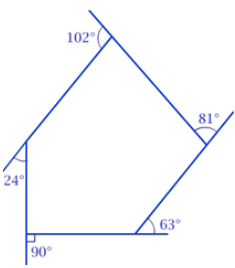Sum of all the exterior angles in the above irregular pentagon is

102° + 81° + 63° +90° + 24° = 360°

Any closed polygon with four sides, four angles and four vertices are known as Quadrilateral. It could be a regular or irregular polygon.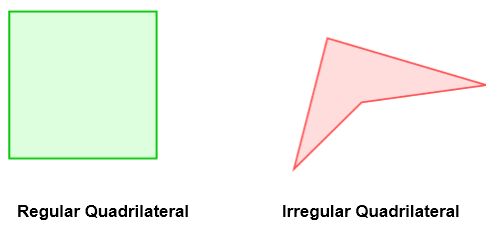Angle sum property of a Quadrilateral

Sum of all the interior angles of a Quadrilateral = 360°

Sum of all the exterior angles of a Quadrilateral = 360°

There are different types of the quadrilateral on the basis of their nature of sides and their angle.

1. Trapezium

If a quadrilateral has one pair of parallel sides then it is a Trapezium.Remark: If the non-parallel sides of a trapezium are equal then it is called Isosceles Trapezium.

2. Kite

If the two pairs of adjacent sides are equal in a quadrilateral then it is called a Kite.Here AB = BC and AD = CD

Properties of a kite

The two diagonals are perpendicular to each other.

One of the diagonal bisects the other one.

∠A = ∠C but ∠B ≠∠D

3. Parallelogram

If the two pairs of opposite sides are parallel in a quadrilateral then it is called a Parallelogram.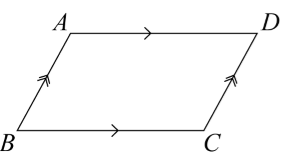Here, AB ∥ DC and BC ∥ AD, hence ABCD is a parallelogram.

Elements of a Parallelogram

Some terms related to a parallelogram ABCD

1. Opposite Sides – Pair of opposite sides are

AB and DC,

2. Opposite Angles – Pair of opposite angles are

∠ A and ∠C

∠B and ∠D

AB and BC

BC and DC

∠A and ∠B

∠B and ∠C

∠C and ∠D

∠A and ∠D

Properties of a Parallelogram

1. The opposite sides of a parallelogram will always be equal.Here, AB = DC and AD = BC.

2. The opposite angles of a parallelogram will always be of equal measure.

As in the above figure, ∠A = ∠C and ∠D = ∠B.

3. The two diagonals of a parallelogram bisect each other.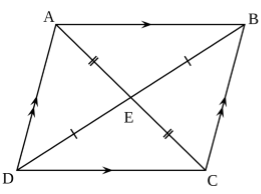Here in ABCD, AC and BD bisect each other at point E. So that AE = EC and DE= EB.

4. The pair of adjacent angles in a parallelogram will always be a supplementary angle.Example

If the opposite angles of a parallelogram are (3x + 5) ° and (61– x) °, then calculate all the four angles of the parallelogram.

Solution

As we know that the opposite angles are equal in a parallelogram so

(3x + 5)° = (61 – x) °

3x + x = 61– 5

4x = 56

x = 14°

Now substitute the value of x in the given angles.

(3x + 5)° = 3(14) + 5

= 42 + 5 = 47°

(61 – x)° = 61 – 14

= 47°

Both the angles are 47° as the opposite angles are equal.

Now to find the other angles let one of the adjacent angles to the above angle is z.

47° + z = 180° (adjacent angles are supplementary angles).

Z = 180° - 47°

= 133°

The fourth angle will also be 133° as the opposite angles are equal.

Hence the four angles of the given parallelogram are 47°, 47°, 133° and 133°.

Some Special Parallelograms

1. Rhombus

If a parallelogram has all the four sides equal then it is called a Rhombus.Here, AB = BC = CD = AD.

Properties of a Rhombus

A rhombus has all the properties of a parallelogram and a kite.

The special property is that its diagonals are perpendicular bisector to each other.

Hence, AE = EC and DE = EB.

It makes an angle of 90° at the point of intersection of the diagonals.

2. Rectangle

A parallelogram with equal angles and equal opposite sides is called Rectangle.Here, AB = DC and AD = BC.

Properties of a Rectangle

Opposite sides are parallel and equal.

Opposite angles are equal.

Adjacent angles make a pair of supplementary angles.

Diagonals are of equal length.

Diagonals bisect each other.

All the four angles are of 90°.

3. Square

A rectangle with all the four equal sides is called a Square.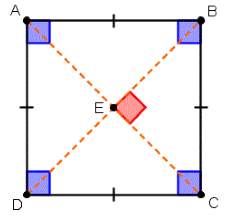Here, AB = BC = CD = AD.

Properties of a Square

All four sides are equal.

Opposite sides are parallel.

Diagonals are of equal length.

Diagonals are perpendicular bisector to each other.

All the four angles are of 90°.

```### Course Features

• 728 Video Lectures
• Revision Notes
• Previous Year Papers
• Mind Map
• Study Planner
• NCERT Solutions
• Discussion Forum
• Test paper with Video Solution